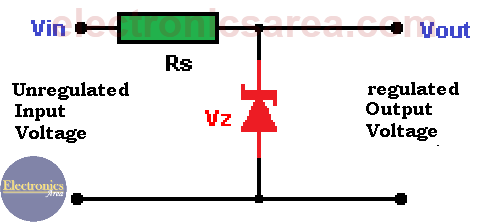# Zener diode voltage regulator circuit

This zener diode voltage regulator circuit uses a Zener diode to establish a reference voltage. This semiconductor is manufactured in a wide variety of voltages and powers. Zener diodes have voltage values from less than 2 volts to several hundred volts, and they can dissipate power ranging from  0.25 to 50 watts… or more.

Using the formula of power Pz = Vz x Iz, you can get the maximum current that can pass through a zener diode: Iz = Pz/Vz. (Amps), where:

• Iz = current through the Zener diode
• Pz = maximum power the zener diode can dissipate (manufacturer data)
• Vz = Zener diode voltage (manufacturer data)

For example: The maximum current that a 10 Volt, 50 Watts zener diode, can endure is Iz = Pz / Vz = 50/10 = 5 amps.

To implement a zener diode voltage regulator, we will use the following diagram.## How to find the resistor (Rs) value?

The calculation of the resistor Rs value is determined by the load current demand (which is connected to this source voltage). Rs is located between “Vin” and the cathode of zener diode. This resistor can be calculated using the formula: Rs = [Vinmin – Vz] /[1.1 x ILmax ], where:

• Vinmin is the minimum value of the input voltage. (Remember that it is an unregulated voltage, and it varies)
• ILmax is the maximum current value that the load may demand.

Once Rs is calculated, the maximum power of this resistor is obtained using the following formula:

PD = [[Vinmin – Vz] / Rs] x Vz

## Design example: Zener Diode Voltage Regulator

A 15 volts unregulated voltage source is used to feed a load that need a 9 VDC. The load requires a current which varies between 200 and 350 mA. (milliamps).

• A 9.1 volts zener diode is chosen (very close to the 9 volts value)
• Rs resistor calculation: Rs = (15 – 9.1) / (1.1 x 0.35) = 15 ohms
• Zener diode power calculation: PD = [(15 – 9.1) / 15] x 9.1 = 3.58 watts.

As there is no 3.58 watts zener diode, we choose a 5 watt diode which is the closest value.

Resistor power calculation

Power is obtained using the formula: P = I2 x R. Replacing data in the formula:

•  Imax = 350 milliamps = 0.35 amps
• Rs = 15 Ohm.

PRs = 0.352 x 15 = 1.84 Watts. You should buy a 2 watt resistor

• 1
•
• 6
•
•
•
•
•
7
Shares
•
7
Shares
• 1
•
• 6
•
•
•
•
Tagged with:

### One Response to “Zener diode Voltage regulator Circuit Design – Diagram”

1.The article is very interesting and helpful. Many thanks